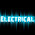## Electronic Devices Objective MCQ Questions with Answers: Part-7

(1) The reverse current in a diode is of the order of
Options:
[a] kA
[b] mA
[c] μA
[d] A
Answers:
1. Options B and C
2. Option C only
3. Option D only
4. Option A and D only

(2) The forward voltage drop across a diode is about....
[a] 2.5V
[b] 3V
[c] 10V
[d] 0.7V

(3) A semiconductor diode is used as
Options:
[a] An amplifier
[b] A Rectifier
[c] An oscillator
[d] A voltage regulator
Answers:
1. Options A and C
2. Option B only
3. A, B, C and D
4. Option D only

(4) A semiconductor diode has ....
[a] One PN junction
[b] Two PN junction
[c] Three PN junction
[d] Four PN junction

(5) A semiconductor diode has forward resistance of order of
Options:
[a] k Ω
[b] Ω
[c] M Ω
[d] μΩ
Answers:
1. Option D only
2. Option B only
3. Options A, B, C and D
4. None of the above

(6) If the arrow of diode symbol is positive with respect to bar, then the diode is .... biased
[a] Forward
[b] Reverse
[c] Either forward or reverse
[d] None of the above

(7) The leakage current in a diode is due to
Options:
[a] Minority Carriers
[b] Majority Carriers
[c] Junction Capacitance
[d] None of the above
Answers:
1. Options A, B, C
2. Option B only
3. Options A and B only
4. Option A only

(8) The DC resistance of a diode is ..... its AC resistance
[a] Same as
[b] More than
[c] Less than
[d] None of the above

(9) An ideal diode is one which behaves as a perfect .... when forward biased
[a] Conductor
[b] Insulator
[c] Resistance material
[d] None of the above

(10) If the temperature of the diode increases,then leakage current....
[a] Remains same
[b] Decreases
[c] Increases
[d] Becomes zero

Please leave your comments below.... Please subscribe to get new posts to your mail ID.....
12:08 AM

#### 4 comments:

1.Nice one sir

2.Thanks
I want transformer related Q/A

3.4.Copied from principles of eletronics text book